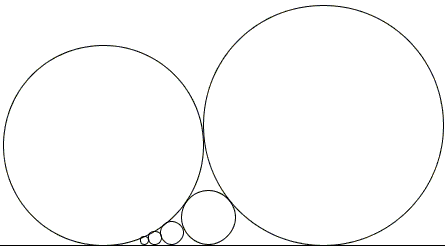## A Sushi Morsel

Twice in his books Ross Honsberger discussed the following problem:

A circle C0 of radius R0 = 1 km is tangent to a line L at Z. A circle C1 of radius 1 mm is drawn tangent to C0 and L, on the right-hand side of C0. A family of circles Ci is constructed outwardly to the right side so that each Ci is tangent to C0, L and the previous circle Ci-1. Eventually the members become so big that it is impossible to enlarge the family further. How many circles can be drawn before this happens?The first solution in [Gems] is rather a brute force use of coordinate geometry. The second solution [Morsels], which Honsberger attributes to C. Stanley Ogilvy, is based on the observation that, if L is seen as the circle of infinite radius, the problem is reduced to the study of the chain of circles in arbelos or as a particular case of Steiner's chains. The problem is then elegantly reduced to the sequence of circles in a strip of paper by inversion in the circle centered at the point of tangency of C0 with L and of radius 2R0.

The earlier discussion comes with no reference; in the later one, Honsberger points to the book Mathematical Games and Pastimes by A. P. Domoryad (Pergamon Press, 1964, problem 19, p. 242).

In a recent article published in The Mathematical Gazette, M. Hajja gives an elementary solution to the problem that makes use of a known result concerning three mutually tangent circles standing on a straight line:Assuming the radii of the three circles in the diagram are successively R0, R1, R2 we have

 (1) 1/√R2 = 1/√R1 - 1/√R0.

By induction, then

 (2) 1/√Rn = 1/√R1 - (n - 1)/√R0.

Denoting 1/Rn = un, we convert (2) to a simple linear relation

 (3) un = u1 - (n-1)u0, n > 1.

Since, for all n, un > 0, (3) shows that the process may continue as long as u1 - (n-1)u0 > 0, o, in other words, while

 (4) n < 1 + u1 / u0, i.e, n ≤ u1 / u0

For R0 = 106mm and R1 = 1 mm, u1 / u0 = 103. Which means that it is impossible to construct circle C1001 and C1000 is the last one, thus solving the problem. (The solution from [Morsels] shows also that R1000 = R0, giving another reason why the process can't continue beyond n = 1000.)

The point of this note is to observe that the problem and the simple solution above both have a long history. Relation (1) is the subject of an 18th century sangaku. Relation (2) appeared in its generality in a 1789 sangaku and in several modifications during the 19th century. This may be one of those cases where Wasan - the traditional Japanese geometry - beat the West to a punch.

### References

1. M. Hajja, On a Morsel of Ross Honsberger, The Math. Gazette, v. 93, n. 527, July 2009, pp. 309-312
2. R. Honsberger, Mathematical Gems, MAA, 1973, pp. 52-53, 153-155
3. R. Honsberger, Mathematical Morsels, MAA, 1978, pp. 218-219## Sangaku